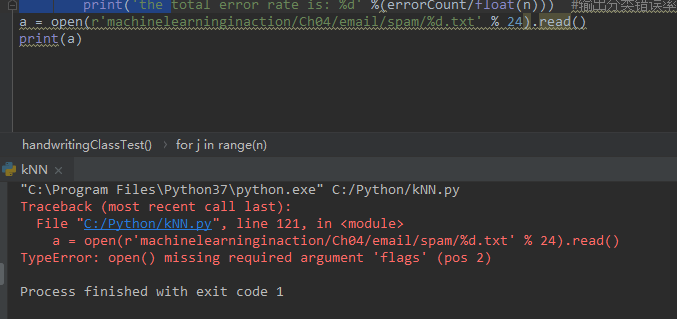Python3的open函数打不开文件，文件的路径没有问题

python3.x再学机器学习中的KNN 用的open函数 老是出错不知道问题在哪2个回答

open("test%d.txt" %(24),"r")with open函数的时候，如何区分什么时候是文件夹 什么时候是文件呢

python使用os.listdir函数的时候的路径问题

python3爬取的内容可以print但是写入本地文件失败，但测试发现写入语句没有问题

# python3 # 源码如下 ``` python import os # 调用系统变量 import re # 正则表达式相关 import urllib import urllib.request import urllib.error import urllib.parse import json import socket import time class ImoocSpider: headers={'User-Agent': 'Mozilla/5.0 (Windows NT 10.0; Win64; x64) AppleWebKit/537.36 (KHTML, like Gecko) Chrome/68.0.3440.106 Safari/537.36'} def getPythonInfo(self,keyWord): myKeyWord = urllib.parse.quote(keyWord) searchUrl='https://www.imooc.com/search/?words='+myKeyWord try: request = urllib.request.Request(url=searchUrl, headers=self.headers) page = urllib.request.urlopen(request) rsp = page.read().decode('unicode_escape') except UnicodeDecodeError as e: print(e) print('-----UnicodeDecodeErrorurl:', searchUrl) except urllib.error.URLError as e: print(e) print("-----urlErrorurl:", searchUrl) except socket.timeout as e: print(e) print("-----socket timout:", searchUrl) else: myres=rsp self.saveFile(myres,keyWord) finally: page.close() print("get_finally") def saveFile(self,res,keyWord): b="./" + keyWord +'.txt' if not os.path.exists(b): # 判断路径指定下是否存在文件/文件夹 try : fp=open(b,'w') print(res,file=fp) #print到文件 except : # print (e) print('文件写入有误') finally : fp.close() print('save_finally') def start (self, keyWord): self.getPythonInfo(keyWord) if __name__ == '__main__': imoocInfo = ImoocSpider() imoocInfo.start('python') ```

python2.7 如何获取下载文件的大小

python2.7 如何获取下载文件的大小 比如说我需要下载 url=https://ask.csdn.net/1.MP4 path=下载路径 我需要获取这个MP4的大小 如果大于50M就不下载 请问用python 怎么实现呢？ 虽然 def callbackfunc(blocknum, blocksize, totalsize): '''回调函数 @blocknum: 已经下载的数据块 @blocksize: 数据块的大小 @totalsize: 远程文件的大小 ''' percent = 100.0 * blocknum * blocksize / totalsize if percent > 100: percent = 100 print "%.2f%%"% percent 可以判断文件大小 但是等你判断的时候 下载已经开始 了

python2 中tkinter 打开文件 中文乱码

python调用一个函数里返回的变量不成功

Python3.7从Entry得到的值在get()之后返回为空

opencv 怎么读取打开的图片

#include <iostream> #include <set> #include <string.h> #include <cmath> #include <cstdlib> #define N 100 using namespace std; struct node { double h,g,f; int x,y; bool operator<(node other)const { return f<other.f; } }; int map[N][N];//迷宫，1表示可以走，0表示不可以走 int row,col;//矩阵的行和列 multiset<node> open;//可以拓展节点 multiset<node>::iterator it; node close[N][N];//已经走过的节点 int step = {1,0,0,1,-1,0,0,-1,1,1,1,-1,-1,1,-1,-1};//走的方式 int prev[N*N];//存放前一个节点 int Init() { memset(close,0,sizeof(close)); } double getg(node a,node b)//获取当前点到终点的路径值 { return sqrt(1.0*(a.x-b.x)*(a.x-b.x)+(a.y-b.y)*(a.y-b.y)); } bool Is_Match(node a,node b) { if(a.x == b.x && a.y == b.y) { return true; } return false; } node begin,end; int main() { while(cin>>row>>col) { open.clear(); Init(); for(int i=0;i<row;i++)//输入迷宫 { for(int j=0;j<col;j++) { cin>>map[i][j]; } } cin>>begin.x>>begin.y>>end.x>>end.y;//起始点和终点 begin.g = getg(begin,end);//初始化起始点的估值函数 begin.f = begin.g; begin.h = 0; open.insert(begin);//open表插入起点 bool IsSuccess = false; while(!open.empty())//但还有节点可以走 { node son = *(open.begin());//取出路径消耗+估值函数最小的节点 open.erase(open.begin());//从open表中删除上面的节点 for(int i=0;i<8;i++)//探寻8个方向 { int u = son.x+step[i]; int v = son.y+step[i]; if(u>=0&&v>=0&&u<row&&v<col&&map[u][v])//如果这个节点在矩阵中，且值为1，可以走 { //更新这个节点的信息，包括路径消耗和估值函数 node tmp; tmp.x = u; tmp.y = v; tmp.g = getg(tmp,end); tmp.h = son.h+1; tmp.f = tmp.h + tmp.g; bool IsInOpen = false;//表示是否在open表中 if(u == end.x&&v == end.y)//如果是终点，就退出循环 { prev[u*7+v] = son.x*7+son.y;//记录前一个节点 IsSuccess = true;//表示成功找到了路径 goto BreakPoint; } for(it = open.begin();it!=open.end();it++) { //如果在open表中，比较tmp这个点与open表中的f的大小，如果前者f小就把tmp插入到open表中 if(Is_Match(*it,tmp)) { IsInOpen = true;//表示在open表中 if((*it).f>tmp.f) { node New = tmp; open.erase(it); open.insert(tmp); prev[tmp.x*7+tmp.y] = son.x*7+son.y;//记录前一个节点 break; } } } bool IsInClose = false;//标记是否在close表中 if(close[tmp.x][tmp.y].f)//如果在close中，表示已经走过，再次比较f的大小，如果前者f小，把这个点插入到open表中，并从close表中删除这个点。 { IsInClose = true;//在close表中 if(tmp.f < close[tmp.x][tmp.y].f) { open.insert(tmp); close[tmp.x][tmp.y].f = 0; close[tmp.x][tmp.y].g = 0; close[tmp.x][tmp.y].h = 0; prev[tmp.x*7+tmp.y] = son.x*7+son.y;//记录前一个节点 } } if(!IsInOpen&&!IsInClose)//如果既不在close表中，也不在open表中，表示没有走过，把这个节点插入open表中。 { open.insert(tmp); prev[tmp.x*7+tmp.y] = son.x*7+son.y;//记录前一个节点 } } } //把从open表中取出点得放到close表中，表示已经走过 close[son.x][son.y].x = son.x; close[son.x][son.y].y = son.y; close[son.x][son.y].g = son.g; close[son.x][son.y].f = son.f; close[son.x][son.y].h = son.h; } BreakPoint:; if(IsSuccess)//如果成功找到了路径，输出路径，我没有去反序输出结果，所以点是倒着的。 { cout<<"The Path Is:"<<endl; int x = end.x*7+end.y; int y = begin.x*7+begin.y; while(x!=y) { cout<<"("<<x/7<<","<<x%7<<") -- > "; x = prev[x]; } cout<<"("<<begin.x<<","<<begin.y<<")"<<endl; cout<<endl; cout<<"Reach Success!"<<endl; } else //不能到达终点 { cout<<"Reach Failed!"<<endl; } } } 这个程序输出的路径是倒着输出的 请问怎么修改才能让它正序输出路径，求帮忙 谢谢

python中使用PIL读取图片，这个图片应事先保存在哪里？

Linux环境下，在Qt中调用Python，导入第三方库时出错？

python中label显示不出图片的问题

python的ctypes加载遇到相互依赖的库如何处理？

python 使用pytesseract出现的问题

import pytesseract import os from PIL import Image pytesseract.pytesseract.tesseract_cmd = 'C:/Program Files (x86)/Tesseract-OCR/tessdata/tesseract.exe' img=Image.open(r'C:/Users/Administrator/Desktop/123.jpg') vcode = pytesseract.image_to_string(img,lang='chi_sim') print vcode 代码很简单，但是执行了之后会报错： File "D:/python_anzhuang/wenjian/pachong3.py", line 9, in vcode = pytesseract.image_to_string(img,lang='chi_sim') File "build\bdist.win32\egg\pytesseract\pytesseract.py", line 294, in image_to_string File "build\bdist.win32\egg\pytesseract\pytesseract.py", line 192, in run_and_get_output File "build\bdist.win32\egg\pytesseract\pytesseract.py", line 107, in save_image File "build\bdist.win32\egg\pytesseract\pytesseract.py", line 99, in prepare TypeError: Unsupported image object 尝试了很多方法都不行，发现一个现象，在pytesseract.py源文件里面随意增加代码对程序本身不影响，所以很好奇执行的pytesseract.py路径在哪里?跪求大神解答，解决可提供报酬。

Python + C/C++ 嵌入式编程：Qt向Python传递数组

loonggg读完需要3分钟速读仅需 1 分钟大家好，我是你们的校长。我之前讲过，这年头，只要肯动脑，肯行动，程序员凭借自己的技术，赚钱的方式还是有很多种的。仅仅靠在公司出卖自己的劳动时...

win10暴力查看wifi密码

!大部分程序员只会写3年代码

HTTP与HTTPS的区别

HashMap底层实现原理，红黑树，B+树，B树的结构原理 Spring的AOP和IOC是什么？它们常见的使用场景有哪些？Spring事务，事务的属性，传播行为，数据库隔离级别 Spring和SpringMVC，MyBatis以及SpringBoot的注解分别有哪些？SpringMVC的工作原理，SpringBoot框架的优点，MyBatis框架的优点 SpringCould组件有哪些，他们...

Python爬虫，高清美图我全都要（彼岸桌面壁纸）